# 2. Selection of lightning rods in the national regulatory documents

The second part of the article "How to choose lightning rods after all?"

Protection zones of rod and catenary wire lightning rods are present in each existing regulatory document: in the "Instruction for lightning protection of buildings and structures AD Industry Standard 34.21.122-87 "  and in the "Instruction for lightning protection of buildings and structures and industrial utilities IS-153-34.21.122-2003" . They are presented there for single rod and catenary wire lightning rods and also for double lightning rods of one type and equal height. The principal difference of these protection zones from those intoduced into the EIC standard is that they originate not fro the top of the lightning rod, but are located lower and thus providing more reliable protection from direct lightning strikes. The main principle of lightning rod operation is considered - increase of protection reliability with the growth of its elevation over the protected object.

Sizes of national protection zones are determined on the base of the so-called statistical method [13,14]. That is why, it is important to understand the need to use the statistical approach to the studied task, set the features of extra-long spark charges, which found their reflection in it and estimate the level of the method formalization, without which it would be hard to use it in applied calculations.

The task about a lightning breakthrough to the protected object is formally brought to the estimation of probability of a puncture of one of intervals of multi-electrode system, simultaneously and paralelly connected to the voltage source. In this case, these intervals have a common high-voltage electrode - channel head of the forming downstream lightning, and the ground surface, lightning rod and the protected object are the electrodes of opposite polarity in the simplest case. The source position of the statistical method is an experimentally fixed possibility of a puncture of any air interval, compatible in length, but not the shortest. It is connected with the fact, that the sparking voltage of each of multi-meter intervals with a sharply unhomogeneous electric field is subject to a significant statistic scatter with the σ deviation standard up to 10% fro the value Up50% corresponding to the 50% probability of a puncture. It is further accepted in the laboratory experiments, that the scatter of physical values of sparking voltages is subject to a normal law with a good precision. For two discharge intervals with average sparking voltages U1 and U2 and σ1 scatter standard, σ2 probability of a puncture of one of gaps, for example the first one, will be equal to the probability of executing of an inequality

For normal distribution of current values of sparking voltages Up2 and Up1, their difference ∆Uр21 is also subject to the normal law, and the average value of this parameter is equal to the difference of average values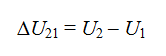(5)

and the σU scatter standard is determined as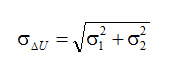(6)

In the result, the probability of a puncture of the sought interval Ф1 can be expressed via a tabulated integral of probability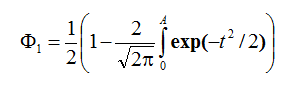(7)

where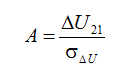(8)

In the sequence of events studied above nothing, except the formal technique of the probability theory is used. All the correlations given are equally applicable for a quantity estimation of probability of any pair of events studied, only if they are carried out independently from each other and the completion of one excludes completion of the other. The statistical method accepts the following for the quantity description of the result of lightning channel strike point selection according to the algorithm given.

In the sequence of events studied above nothing, except the formal technique of the probability theory is used. All the correlations given are equally applicable for a quantity estimation of probability of any pair of events studied, only if they are carried out independently from each other and the completion of one excludes completion of the other. The statistical method accepts the following for the quantity description of the result of lightning channel strike point selection according to the algorithm given:

1. Having started from a thunder cloud, lightning channel is first formed without any reaction for the state of the ground surface and grounded structures located on it. It happens until the head of the lightning channel goes down to some height, which is called height of Ho orientation. IN any place on the orientation flat, the probability of channel head location is equally probable.
2. Starting from level Ho, the process of orientation begins, which defines the probability of a lightning development to the system lightning rod-object, but not to the undisturbed ground surface. The formation of plasma channels in these two principally different directions can be studied without their mutual influence and that is why, the mathematical formalism can be fully used for the description of probability of these events (4)-(8)
3. Exclusively great length of discharge intervals from the orientation flat to the ground and to the top of the lightning rodat a first approximation allows to accept equal average values of electric strength Епр. It gives a chance to get rid of certain values of electric strengths in (5) - (6) and operate only with geometric sizes of discharge intervals to the ground surface Sз = Ho and to the top of the lightning rod of height  hm (fig. 6).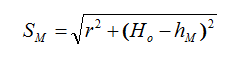(9)

In the result, the upper limit of the probability integral in (7) can be presented as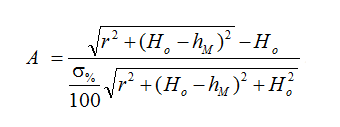(10)

here r - radialoffset of the lightning channel head in the plane of orientation relatively to the vertical axis of the lightning rod, σ% - standard of scatter of sparking voltage of isolated multi-meter interval, expressed in percentage from Up.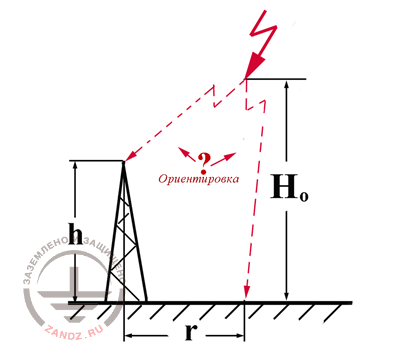Fig. 6 To the estimation of lightning orientation probability

Ориентировка - orientation

At that, the orientation probability is preseted in the form of the introduced probability integral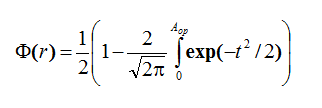(11)

4. As already mentioned, the choise of strike point on the surface of lightning rod and the protected object, is carried out at the expense of the competing development of counter leaders from their tops. This process, also statistical in all physical development, is significantly more determined. The reason is that some counter leader, which occasionally exceeds the development of the other, weakens the electric field by its spacial charge in the place of location of leader channels lagging behind, and thus, delaying their growth in a greater extent. Detailed laboratory research in long intervals showed [2,3,13] that the effect of positive feedback appearing this way, can be formally displayed in the frames of the algorithm, offered for the calculation of orientation process, if introduce a new statistic parameter instead of σ scatter standard - standard of σвыб choice, the value of which should be the lower σ, the closer the ligthtning rod and the protected object are located to each other. In the result, it becomes possible to fix a calculation expression for a probability of a strike point choice on the protected object, which is similar in its structure (10) - (11).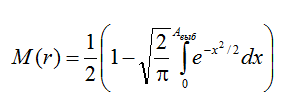(12)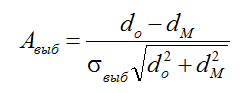(13)

Here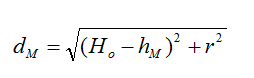(14)

is the shortest distance from the head of the lightning channel at the orietation height to the top of the lightning rod oh hM height;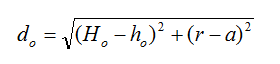(15)

similar distance to the object with the height ho at the distance between it and the lightning rod, equal to a.

Lightning, position of the head of which in the orientation place is characterized by the coordinate r, will breakthrough to the protected object with the probability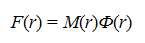(16)

At that, in order to define the full number of breakthroughs it is enough to integrate F(r) along the orientation plane, multiplying the integration result to the relative density of lightning discharges on the studied territory nM: in case of radial symmetry of the system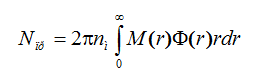(17)

The algorithm, similar to the one presented, can be distributed to an arbitrary number of lightning rods and objects of various configuration and height, if to operate with shortest distances from the lightning channel head, at each calculation stage, with the fixed coordinates in the orientation plane to the stufied system of lightning rods and to the set of protected objects, and hold the orientation in (17) according to Descarter's coordinates. Expression (17) allows to calculate both the full number of lightning strikes into the studied system of lightning rods and the protected objects on the whole, if to put M=0. The latter also gives the estimation of a probability of a lightning breakthrough to the protected object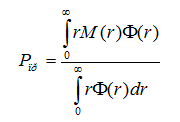(18)

In the algorithm given, there are three parameters, except the geometrical sizes of onground structures, which in certain extent can be studied as adjustable: standard of σ scatter and σвыб and height of Ho orientation. The latter parameter undoubtedly depends on the charge value, which is transported to the ground by the downstream lightning channel (and on its current, consequently). The specialists still do not have the damage statistics of on-ground structures by lightning of difference force, and that is why, the differentiation of orientation height for lightning with different currents is not authentic yet. An average value of orientation height can be estimated coming out from the average value of lightning contraction radius Ratt, which for an object of height h, as a rule, is taken equal to  Ratt= 3h. And the orientation height in this case is defined as the equality of distances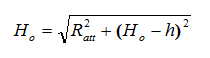(19)

Where Ho = 5h. This value was used in the next calculations. Relative value of σ=0,1 standard is extracted from the experiments with extremely long laboratory intervals. Its precision doesn't have big sense, because the calculation results are weakly sensitive to this parameter.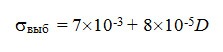(20)

E. M. Bazelyan, DEA, professor
Energy Institute named after G.M. Krzyzanowski, Moscow

Read more "3. Possibilities of the statistical method"